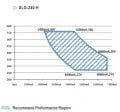# Sizing power supply for multiple led drivers

#### jeffkrol

Joined Dec 8, 2015
42
I need to be more exact in sizing a power supply when I'm using multiple drivers.
Here is the scenario:
56V DC output power supply.
8 led drivers in parallel with multiple voltages on them but all at constant current.
DC/DC step down drivers
List:
1) 52v * .7A = 36.4W
2) 52 * .7 = 36.4
3) 52 * .7 = 36.4
4) 52 * .7 = 36.4
5) 17.6 * .7 = 12.32
6) 25.6 * .7 = 17.92
7) 8.8 * .7 = 6.16
8) 26.4 * .7 = 18.48

200.48 Total Watts.

What is the amperage rating needed on the 56V power supply?

I usually just take .7 x 8 = 5.6 Amp capable power supply but that is the easy
way and I don't believe it is correct. Just insures me I don't under supply it.. I think.

See I was looking at a Meanwell 240 watt CC/CV power supply that had a "Recommended performance region"
like this:4.28A @ 56V

Oh I'm tempted just to use the Hep-320-54 but it is 2x the cost of the above.
49-58V
2.97- 5.95A

What do you think?
Point is I need to know the RIGHT way to look at this.
Another fact: Driver needs a v differential of about 4V so ps needs
to be 52+4 V capable
Thanks!

Not considering efficiency atm.

Last edited:

#### Irving

Joined Jan 30, 2016
3,417
If you are deriving the lower voltages in elements 5 - 8 with decent efficiency buck converters you need to assume 10-15% conversion loss. In which case your load is:

4 × 36.4 + ( 12.32 + 17.92 + 6.16 + 18.48 ) ÷ 0.85 = 210.2W​
= 56v @ 3.8A​
The XLG-240-H is perfectly suitable.

•jeffkrol